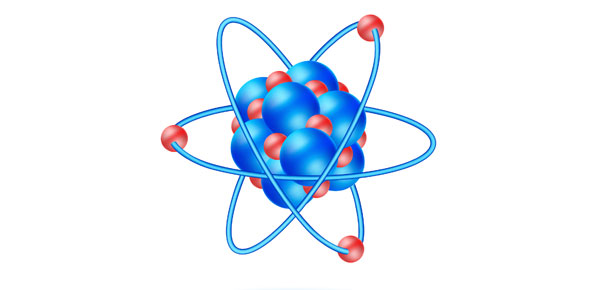# Physical Science Machines And Energy

22 Questions | Attempts: 81SettingsPhysical Science Quiz

• 1.
Speed is the rate at which an object_______
• 2.
What is Velocity?
• A.

Spped

• B.

Speed in a given direction

• C.

Speed in a given distance

• D.

Direction in a given speed

• 3.
The rate of_______in ___________ is Acceleration
• 4.
All moving objects have________-
• A.

Momentum

• B.

Mass

• C.

Velocity

• 5.
A force is a________
• 6.
The first law of motion is also called.....
• 7.
The first law of motion states...
• A.

That an object at rest will remain at rest and an object in motion will remain in motion at constant velocity untill acted upon by an unbalenced force.

• B.

Shows how force, mass, and acceleration are related.

• C.

That for every action, there is an equal and opposite reaction.

• 8.
The second law of motion states......
• A.

That for every action, there is an equal and opposite reaction.

• B.

That an object at rest will remain at rest and an object in motion will remain in motion at constant velocity untill acted upon by an unbalenced force.

• C.

Shows how force, mass, and acceleration are related.

• 9.
The third law of motion states.....
• A.

That an object at rest will remain at rest and an object in motion will remain in motion at constant velocity untill acted upon by an unbalenced force.

• B.

Shows how force, mass, and acceleration are related.

• C.

That for every action, there is an equal and opposite reaction.

• 10.
Gravity equals.......
• A.

9.8 meters per second squared

• B.

8.8 meters per second squared

• C.

9.8 meters per secong cubed

• D.

1000000000000000000000000000000000000mps

• 11.
Weight is a measure of the___________
• 12.
The "Push" or force particles exert over a certain area is called___
• 13.
Power is the rate at which work is_________
• 14.
Describe what work input is and what work output is? How are they different?
• 15.
An instrumenthat makes work easier is called a______
• 16.
The comparison of work output to work input is called the_______
• 17.
How many types of simple machines are there?
• A.

5

• B.

6

• C.

7

• D.

8

• E.

9

• 18.
The machines are_____
• A.

The wedge, the screw, the lever, the pulley, the wheel and axle ,and the inclined plane.

• B.

The wheel, the saw, the axle, the simple machine, the lever, the pulley

• C.

The pulley, the wheel and axle, the screw, the lever, the inclined plane, the ax

• 19.
How many forms of enegy are there?
• A.

2

• B.

3

• C.

4

• D.

5

• E.

6

• 20.
Which are the forms of energy?
• A.

Mechanical Energy

• B.

Heat Energy and Chemical Energy

• C.

Electromagnetic Energy

• D.

Nuclear Energy

• E.

All of the above

• 21.
Kinetic Energy is energy in____________ Potential energy is energy of_____________
• 22.
The law of conversion states......

## Related TopicsBack to top
×

Wait!
Here's an interesting quiz for you.